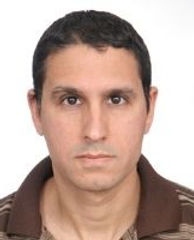top of page
###### Fields of Research###### School of Computer Science
• Number Theory

• Field Theory

• General Algebra

## Short Bio

Dr. Boaz Cohen received a Ph.D. In mathematics from the University of Tel Aviv in 2015, and he is currently a faculty member in the Academic college of Tel Aviv and an associate faculty member in the University of Tel Aviv, where he enjoys teaching all levels of undergraduate's mathematics.

## Selected Publications

1. Cohen B., Chebyshev polynomials and Pell equations over finite fields. To appear in Czechoslovak Mathematical Journal.

2. Cohen B., The structure of the n-th roots of unity in Residue rings of prime ideals P over p in Algebraic Number Fields. Part IV: n-th roots of unity for general n, International Mathematical Forum, 13 (4), 161-174, January 2018.

3. Cohen B., The structure of the n-th roots of unity in Residue rings of prime ideals P over p in Algebraic Number Fields. Part III: n-th roots of unity when n=p^b, International Mathematical Forum, 13(1):15-64, January 2018.

4. Cohen B., The structure of the n-th roots of unity in Residue rings of prime ideals P over p in Algebraic Number Fields. Part II: n-th roots of unity when P||(p), International Mathematical Forum, 12:457-468 · January 2017.

5. Cohen B., The structure of the n-th roots of unity in Residue rings of prime ideals P over p in Algebraic Number Fields. Part I: n-th roots of unity when p does not divide n, International Mathematical Forum, 12: 439-455, January 2017.

6. Cohen B., Generalized Pell Equations for 2 × 2 Matrices, Discussiones Mathematicae, General Algebra and Applications, 37 (2017) 13–30.

7. Cohen B., Generalization of the Hall-Witt identity, Isr. J. Math. (2017) 221: 605.

bottom of page